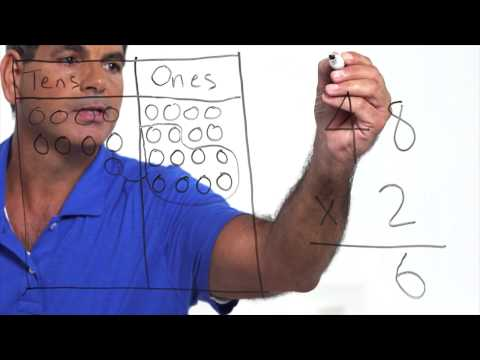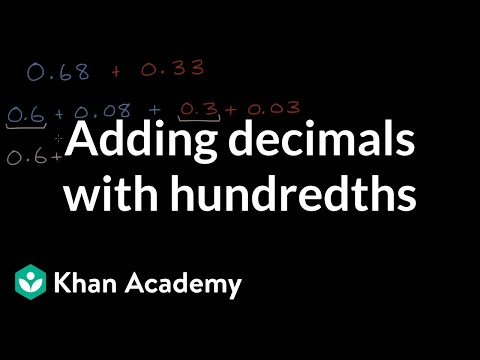Video

# Subtracting decimals: 10.1 - 3.93 (Full video)

Description: Sal subtracts 10.1 - 3.93 using the standard algorithm. Created by Sal Khan. Let's try to calculate 10.1 minus 3.93. So let's just rewrite it, aligning the decimal and the different place values. Now, let's just try to calculate this. Now, before we subtract, we want all the numbers on top to be larger than the numbers on the bottom. And we don't even have a number here. We could stick a 0.

### Other videos you might be interested in### Math Studio Talk: Instruction for 5.NBT (Full video)

#### EngageNY